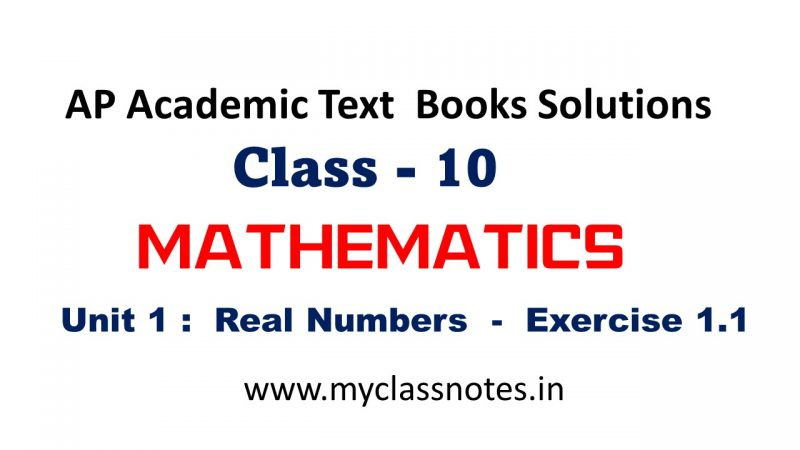Select Page

Real Numbers Exercise 1-1 , New Complete answers – real numbers from class 10  Most imp questions. Its for SSC Public exams and also for competitive exams  like NTSE, RGUKT CET, POLYCET, APRJC etc…Real Numbers Exercise 1-1

“God made the integers. All else is the work of man”…….…. Leopold Kronecker
Euclid’s division lemma: Given positive integers a, b there exists unique pair of integers q and r satisfying
a = bq +r; 0≤r<b
This result was first published / recorded in book VII of Euclid’s “The Elements”  Euclid’s division algorithm is a technique to compute the Highest Common Factor
(H.C.F) of two given numbers.
E.g: HCF of 80 and 130
130 = 80 X 1 + 50
80 = 50 x 1 + 30
50 = 30 X 1 + 20
30 = 20 × 1 + 10
20 = 10 x 2 + 0 and H.C.F = 10

### Real Numbers Exercise 1-1

Euclid’s division algorithm can also be extended to all integers. Numbers which can be expressed in the form p/q, where q # 0 and ‘p and q’ are
integers are called rational numbers; represented by Q.
Q={ p/q ; q is not equal to 0, p,q ∈ Z}
Every rational number can be expressed either as a terminating decimal or as a non-terminating recurring decimal.
Numbers which can’t be expressed in algebraic form i.e in p/q form are called irrational numbers represented by S. You may notice that the first letter of surds is ‘S’

The combined set of rationals and irrationals is called the set of Real numbers; represented by R
R= QUS.